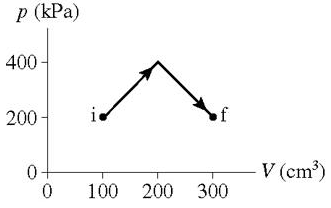# Problem: How much work is done on the gas in the process shown in the figure?

###### FREE Expert Solution

Work done ON a gas:

$\overline{){\mathbit{W}}{\mathbf{=}}{\mathbf{-}}{\mathbit{P}}{\mathbf{·}}{\mathbf{∆}}{\mathbit{V}}}$

$\overline{){\mathbf{1}}{\mathbf{Pa}}{\mathbf{=}}{\mathbf{1}}{\mathbf{N}}{\mathbf{/}}{{\mathbf{m}}}^{{\mathbf{2}}}}$

For an expansion work done is negative while for compression work done is positive.

The change in volume form i to f represents an expansion. Thus, the work done is negative.

To get the magnitude of the work done we'll sum the area of the shapes (rectangle and triangle) under the curve.

81% (350 ratings)###### Problem Details

How much work is done on the gas in the process shown in the figure?